# Free Cash Flow to Firm (FCFF)

The amount of cash available to holders of a company's equity and debt

Free cash flow to the firm (FCFF) is the amount of cash available to holders of a company's equity and debt after accounting for expenses, taxes, and investments. It is equal to a company's cash flow from operations (CFO) minus any capital expenditures (CapEx).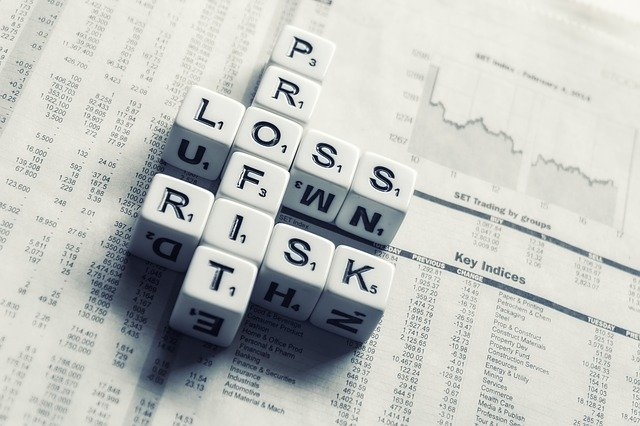It is often used in discounted cash flow (DCF) valuations to get to the intrinsic value of a company. The cash flow statement contains the necessary variables to calculate FCFF.

If the cash flow statement isn't available, the income statement and balance sheet can be used to calculate a company's FCFF by using net income and making adjustments for non-cash items (depreciation) and changes in working capital

A company may use free cash flow to invest in new projects or to pay dividends to its shareholders.

Why Is Free Cash Flow Important?

It is essential because it is the cash flow available to all providers of a firm's capital, i.e., when a firm issues bonds, the investors give it money in exchange for bonds.

It is also used as a measure of creditworthiness. Generally, companies with higher FCFFs are more likely to qualify for better debt or equity financing options with lower costs, thus attracting shareholder interest.

It is also an indicator that a company will generate sufficient cash flows to satisfy its current and future obligations and potentially pay extra money to shareholders.

Free Cash Flow To The Firm Formula

The formula is as follows:

FCFF = CFO - CapEx

where,

• CFO: Cash flow from operations

• CapEx: Capital expenditures

Below we will go into greater detail about what makes up CFO and CapEx.

## Cash Flow From Operations (CFO) Calculation

Since cash flow from operations already includes non-cash adjustments, such as depreciation and changes in working capital, the formula for free cash flow to the firm usually begins with cash flow from operations (CFO), which can be found on the cash flow statement

If the cash flow statement isn't available, CFO can be derived by starting with net income. However, it is important to remember that cash flow from operations (CFO) is not the same as free cash flow, but it is part of the free cash flow formula.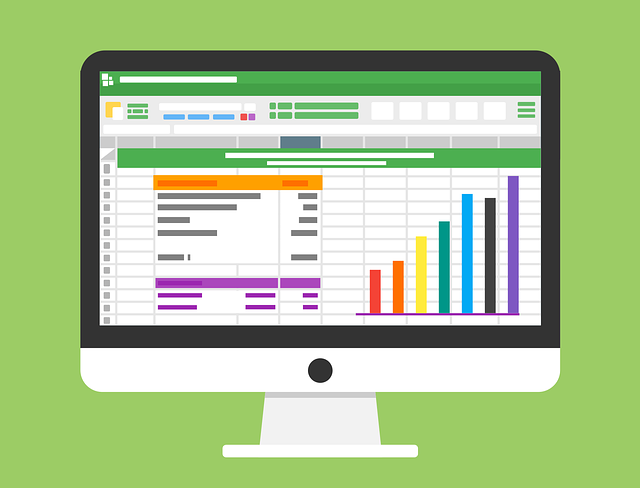If starting with net income, adjustments will need to be made for non-cash items and changes in working capital.

Cash flow from operations represents the amount of cash that a company generates from its normal operating activities; for instance, the total cost and sales of Apple's iPhone would be included in Apple's cash flow from operations.

The formula for cash flow from operations is below.

CFO = Net Income + Non-Cash Adjustments + Changes In Working Capital

where,

Let's use a real-world example and calculate the cash flow from operations for Apple Inc as of September 2021.

Below is a step-by-step example of the calculation.

• Depreciation, Depletion, & Amortization of \$11.28

• Deferred Taxes & Investment Credit: -\$4.77

• Other Funds: \$7.76

Step 3: Add changes in working capital for the period of -\$4.91

• Receivables: -\$14.03

• Inventories: -\$2.64

• Accounts Payable: \$12.33

• Other Assets/Liabilities: -\$.57

Step 4: Calculate a CFO of \$104.4 billionPlease look at the video below from our Financial Statement Modeling Course to better understand the concept.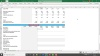## Capital Expenditures (CapEx) Calculation

Capital expenditures (CapEx) are the amount of money a company spends on maintaining existing physical assets, such as factories or machinery, and new physical assets, such as new land or equipment.

It does not include costs from normal operations, such as utility payments or cleaning services.

Physical assets include:

• Property

• Machinery

• Factories and warehouses

CapEx can be found on the cash flow statement and is categorized as investing activities or cash flow from investing (CFI).

Below is the formula for capital expenditures (CapEx):

CapEx = Change in PP&E + Depreciation/Amortization

where,

• Change in PP&E: Current property, plant, and equipment minus prior property, plant, and equipment. PP&E is on the balance sheet.

• Depreciation/Amortization: Depreciation, amortization, and depletion are on the income statement.

Capital expenditures for Apple in 2021 were equal to \$11.09 billion.

## Free Cash Flow to the Firm Calculation

We can now calculate Apple's free cash flow to the firm using Apple's CFO and CapEx.

The formula is:

FCFF = CFO - CapEx

where,

CFO: Net Income + Non-Cash Adjustments + Changes In Working Capital

CapEx: Change in PP&E + Depreciation/Amortization

CFO for Apple as of September 2021 was equal to \$104.4 billion, and CapEx for the same period was \$11.09 billion.

Using the formula, we get \$92.95 billion (104.4 - 11.09).## Calculating Free Cash Flow Using Net Income, EBITDA, And EBIT

The starting point for calculating free cash flow will vary depending on the availability of the cash flow statement.

If the cash flow statement is available, the starting point will be a firm's cash flow from operations (CFO). If the cash flow statement isn't available, the income statement and balance sheet may be used to calculate CFO using net income as the starting point.

We will discuss how to arrive at free cash flow using net income, EBITDA (earnings before interest, taxes, depreciation, and amortization), and EBIT (earnings before interest and taxes) in the following section.

## Free Cash Flow To The Firm From Net Income

Net income will be the starting point to calculate free cash flow if the cash flow statement isn't available to use CFO. Net income is the last line item on the income statement.

Below is the income statement for Apple Inc.

If net income is used, adjustments for non-cash items (depreciation, amortization, deferred tax items) and changes in working capital must be made to calculate free cash flow.

Interest expense must be added back because interest paid to debt holders is often tax deductible (reduces taxes payable), so the interest paid is multiplied by the corporate tax rate to capture the interest that will be deductible.

The formula when using net income as the starting point is provided below:

FCFF = Net Income + Non-Cash Adjustments + Interest Expense - Changes In Working Capital - CapEx

where,

• Net Income: Revenues minus expenses

• Non-cash adjustments: Depreciation, amortization, deferred tax items

• Interest expense: Interest Paid (Tax Rate)

• Changes in Working Capital: Current assets - Current liabilities

• CapEx: Capital Expenditures

## Free Cash Flow To The Firm From EBITDA

Earnings before interest, taxes, depreciation and amortization (EBITDA) may be used as the starting point to calculate free cash flow. If EBITDA is used, we need to adjust it for taxes, and because depreciation is often tax-deductible, we must add back the depreciation that is tax-deductible.

Below is the formula for when using EBITDA:

FCFF = EBITDA(1 – Tax rate) + Depreciation(Tax rate) – CapEx

where,

• EBITDA: Earnings before interest, taxes, depreciation, and amortization

• Tax Rate: Corporate tax rate

• CapEx: Capital expenditures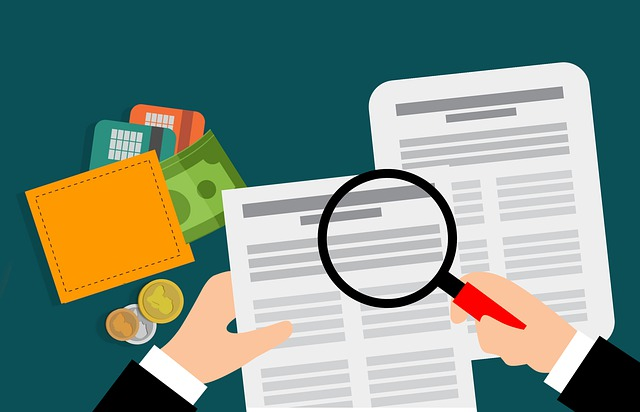## Free Cash Flow To The Firm From EBIT

Earnings before interest and taxes (EBIT) can also be used as the starting point to calculate free cash flow.

Similar to when using EBITDA as the starting point, adjustments must be made to add back depreciation, since it is tax-deductible, and adjust EBIT for taxes.

Below is the formula when using EBIT:

FCFF = EBIT(1 – Tax rate) + Depreciation – CapEx

where,

• EBIT: Earnings before interest and taxes

• Tax Rate: Corporate tax rate

• CapEx: Capital expenditures

# Free Cash Flow To The Firm: Levered Vs. Unlevered

Levered free cash flow (LFCF) is the amount of cash available to pay dividends to shareholders, stock repurchases, or reinvestment. It is the amount of money available after a company has spent all its expenses, including debt obligations (payments to bondholders).

In other words, it is the amount of money available to pay holders of a company's equity (stock).

Levered free cash flow is essential because it is the firm's equity value, i.e., what the company is worth to equity investors.

Below is the formula for levered free cash flow:

LFCF = CFO - CapEx - D

where,

• CFO: Cash flow from operations

• CapEx: Capital Expenditures

• D: Debt principal repayments

Unlevered free cash flow (UFCF) is the cash available after all expenses are paid but before debt obligations are paid. In other words, it is the amount of money available to pay both holders of a company's equity and debt.

Unlevered free cash flow is important because it values the company's core business operations. It is the enterprise value (EV) of the firm.

Below is the formula for unlevered free cash flow:

UFCF = EBIT(1-TR) + Non-cash Charges- CapEx - Changes in Working Capital

where,

• EBIT: Earnings before interest and taxes

• TR: Marginal tax rate

• Non-cash Charges: Depreciation, amortization, deferred tax items

• CapEx: Capital Expenditures

• Working Capital: Current assets (Inventory, accounts receivable, and cash) minus current liabilities (accounts payable and debt)

Please look at the following video from our DCF Modeling Course to learn more about the difference between levered and unlevered free cash flow.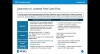# Free Cash Flow To The Firm Vs. Free Cash Flow To Equity

Free cash flow to the firm is cash available to a company's equity and debt holders. Free cash flow to equity (FCFE) is cash available only to holders of a company's equity (common shareholders).

Free Cash Flow To The Firm Vs. Free Cash Flow To Equity
Free Cash Flow To The FirmFree Cash Flow To Equity
Cash available to bondholders, common stockholders, and preferred stockholdersCash available to common stockholders

It is equal to a company's cash flow from operations (CFO) minus any capital expenditures (CapEx).

FCFF = CFO - CapEx

where,

• CFO: Cash flow from operations

• CapEx: Capital Expenditures

Free cash flow to equity can be derived in two different ways: one using CFO as the starting point, and the second using FCFF. Both equations are provided below.

FCFE equals the company's cash flow from operations (CFO) minus CapEx plus net borrowing (debt issued minus debt repaid).

FCFE = CFO - CapEx + Net Borrowing

where,

• CFO: Cash flow from operations

• CapEx: Capital Expenditures

• Net Borrowing: Debt issued minus debt repaid in the current period

If you notice in the above equation, (CFO - CapEx) is equal to FCFF; thus, if it has already been calculated: FCFE can be derived from it by adding net borrowing to FCFF.

FCFE = FCFF + Net Borrowing

where,

• FCFF: Free cash flow to the firm

• Net Borrowing: Debt issued minus debt repaid in the current period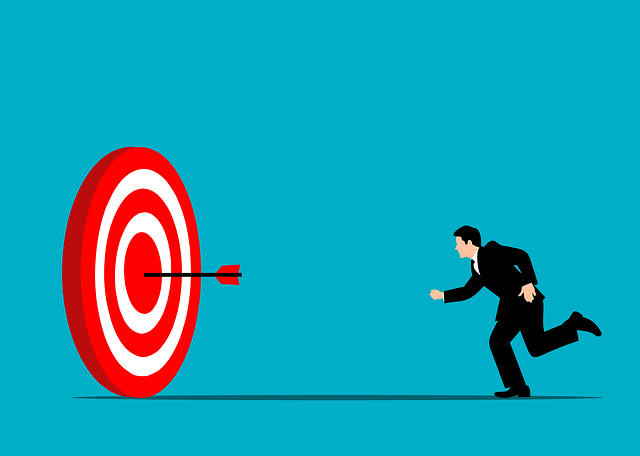1. Discounting Free Cash Flow

When calculating the intrinsic value of a company, the discount rate that is used will differ depending on the numerator, FCFF or FCFE, that is used. Below we will explain discounting free cash flow to the firm and free cash flow to equity.

2. Discounting Free Cash Flow To The Firm

It is the total cash flow available to all holders of a company's equity and debt, so when discounting free cash flow to find the intrinsic value of a company, the weighted average cost of capital (WACC) is used as the discount rate.

The weighted average cost of capital is the after-tax required rate of return for holders of a company's equity and debt. So using WACC to discount FCFF provides the entire capital value of a company's available capital.

Below is the formula to find the intrinsic value of a firm using free cash flow to the firm.where,

• FCFF: Free cash flow to the firm

• WACC: Weighted average cost of capital

• t: current period, input is usually "1"

• t+n: first period plus the next period, for instance, if the first period (t) is 1, (t+n) will equal 2 (1+1)

Once the firm's value is calculated, a firm's equity value can be calculated by subtracting the market value of a company's debt.

Equity Value = Value Of The Firm - Market Value of Debt

## Discounting Free Cash Flow to Equity

Free cash flow to equity is the amount of cash available to the holders of a company's common stock. When discounting free cash flow to equity, the cost of equity is used as the discount rate to get the value of the company's equity.

The cost of equity is the required rate of return required by holders of a company's common stock.

Below is the formula to find the value of a firm's equity.where,

• FCFE: Free cash flow to equity

• r: Cost of equity

• t: current period, input is usually "1"

• t+n: first period plus the next period, for instance, if the first period (t) is 1, (t+n) will equal 2 (1+1)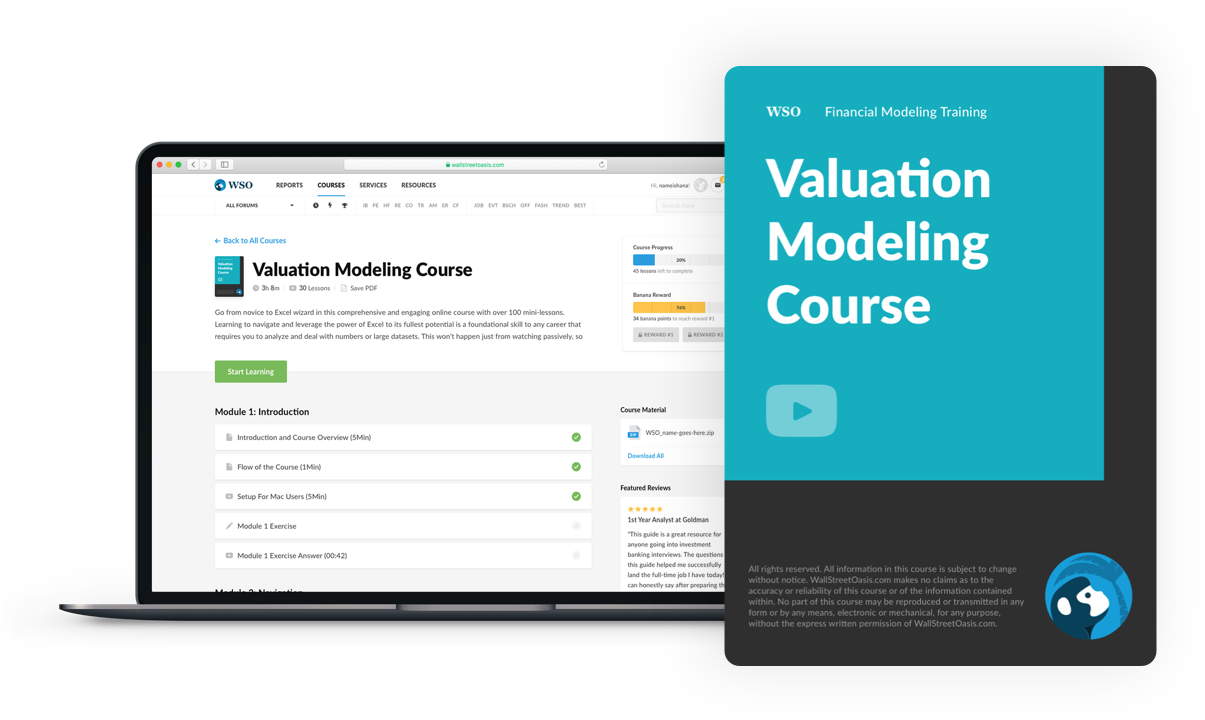### Everything You Need To Master Valuation Modeling

To Help You Thrive in the Most Prestigious Jobs on Wall Street.

Researched & Authored by Ryan